Which of the adjoining graphs represents ohmic resistance :

(1)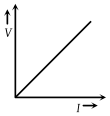(2)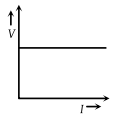(3)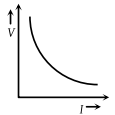(4)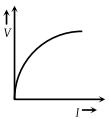Concept Questions :-

Derivation of Ohm's law
High Yielding Test Series + Question Bank - NEET 2020

Difficulty Level:

Variation of current passing through a conductor as the voltage applied across its ends as varied is shown in the adjoining diagram. If the resistance (R) is determined at points A, B, C and D, we will find that :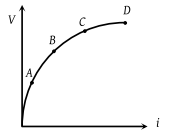(1) RC = RD

(2) RB > RA

(3) RC > RB

(4) None of these

Concept Questions :-

Derivation of Ohm's law
High Yielding Test Series + Question Bank - NEET 2020

Difficulty Level:

The voltage V and current I graph for a conductor at two different temperatures T1 and T2 are shown in the figure. The relation between T1 and T2 is :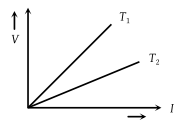(1) T1 > T2

(2) ${T}_{1}\approx {T}_{2}$

(3) T1 = T2

(4) T1 < T2

Concept Questions :-

Derivation of Ohm's law
High Yielding Test Series + Question Bank - NEET 2020

Difficulty Level:

From the graph between current I and voltage V shown below, identify the portion corresponding to negative resistance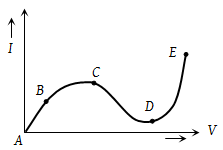(1) AB

(2) BC

(3) CD

(4) DE

High Yielding Test Series + Question Bank - NEET 2020

Difficulty Level:

I-V characteristic of a copper wire of length L and area of cross-section A is shown in figure. The slope of the curve becomes :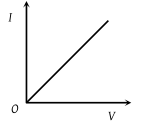(1) More if the experiment is performed at higher temperature

(2) More if a wire of steel of same dimension is used

(3) More if the length of the wire is increased

(4) Less if the length of the wire is increased

Concept Questions :-

Derivation of Ohm's law
High Yielding Test Series + Question Bank - NEET 2020

Difficulty Level:

E denotes electric field in a uniform conductor, I corresponding current through it, Vd drifts velocity of electrons and P denotes thermal power produced in the conductor, then which of the following graph is incorrect :

(1)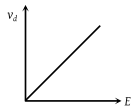(2)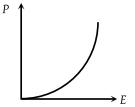(3)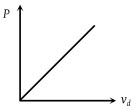(4)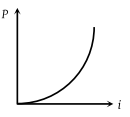Concept Questions :-

Current and current density
High Yielding Test Series + Question Bank - NEET 2020

Difficulty Level:

The two ends of a uniform conductor are joined to a cell of e.m.f. E and some internal resistance. Starting from the midpoint P of the conductor, we move in the direction of current and return to P. The potential V at every point on the path is plotted against the distance covered (x). Which of the following graphs best represents the resulting curve

(1)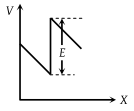(2)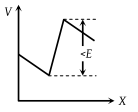(3)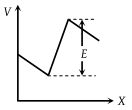(4)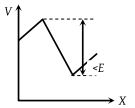Concept Questions :-

Emf and terminal voltage
High Yielding Test Series + Question Bank - NEET 2020

Difficulty Level:

The resistance Rt of a conductor varies with temperature t as shown in the figure. If the variation is represented by ${R}_{t}={R}_{0}\left[1+\alpha t+\beta {t}^{2}\right]$, then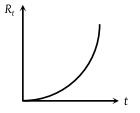(1) α and β are both negative

(2) α and β are both positive

(3) α is positive and β is negative

(4) α is negative and β are positive

Concept Questions :-

Derivation of Ohm's law
High Yielding Test Series + Question Bank - NEET 2020

Difficulty Level:

Variation of current and voltage in a conductor has been shown in the diagram below. The resistance of the conductor is :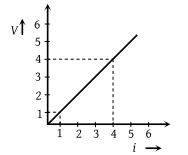(1) 4 ohm

(2) 2 ohm

(3) 3 ohm

(4) 1 ohm

Concept Questions :-

Derivation of Ohm's law
High Yielding Test Series + Question Bank - NEET 2020

Difficulty Level:

Resistance as shown in figure is negative at(1) A

(2) B

(3) C

(4) None of these

Concept Questions :-

Derivation of Ohm's law